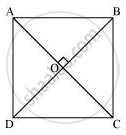# Show that If the Diagonals of a Quadrilateral Are Equal and Bisect Each Other at Right Angles, Then It is a Square. - Mathematics

Show that if the diagonals of a quadrilateral are equal and bisect each other at right angles, then it is a square.

#### SolutionLet us consider a quadrilateral ABCD in which the diagonals AC and BD intersect each other at O. It is given that the diagonals of ABCD are equal and bisect each other at right angles. Therefore, AC = BD, OA = OC, OB = OD, and ∠AOB = ∠BOC = ∠COD = ∠AOD = 90º. To prove ABCD is a square, we have to prove that ABCD is a parallelogram, AB = BC = CD = AD, and one of its interior angles is 90º.

In ΔAOB and ΔCOD,

AO = CO (Diagonals bisect each other)

OB = OD (Diagonals bisect each other)

∠AOB = ∠COD (Vertically opposite angles)

∴ ΔAOB ≅ ΔCOD (SAS congruence rule)

∴ AB = CD (By CPCT) ... (1)

And, ∠OAB = ∠OCD (By CPCT)

However, these are alternate interior angles for line AB and CD and alternate interior angles are equal to each other only when the two lines are parallel.

∴ AB || CD ... (2)

From equations (1) and (2), we obtain

ABCD is a parallelogram.

In ΔAOD and ΔCOD,

AO = CO (Diagonals bisect each other)

∠AOD = ∠COD (Given that each is 90º)

OD = OD (Common)

∴ ΔAOD ≅ ΔCOD (SAS congruence rule)

∴ AD = DC ... (3)

However, AD = BC and AB = CD (Opposite sides of parallelogram ABCD)

∴ AB = BC = CD = DA

Therefore, all the sides of quadrilateral ABCD are equal to each other.

AC = BD (Given)

DC = CD (Common)

∴ ΔADC ≅ ΔBCD (SSS Congruence rule)

∴ ∠ADC = ∠BCD (By CPCT)

However, ∠ADC + ∠BCD = 180° (Co-interior angles)

One of the interior angles of quadrilateral ABCD is a right angle.

Thus, we have obtained that ABCD is a parallelogram, AB = BC = CD = AD and one of its interior angles is 90º. Therefore, ABCD is a square.

Concept: Another Condition for a Quadrilateral to Be a Parallelogram
Is there an error in this question or solution?
Chapter 8: Quadrilaterals - Exercise 8.1 [Page 146]

#### APPEARS IN

NCERT Class 9 Maths